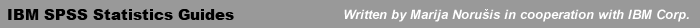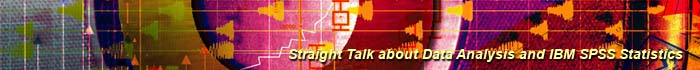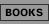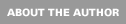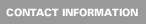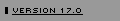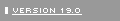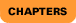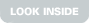SPSS 17.0 Statistical Procedures Companion: Chapters

 Introduction. An overview of the statistical procedures described in the book. Getting to Know SPSS. Tutorials; windows; dialog boxes; the Help system; Pivot Table Editor; Chart Editor; using command syntax. Introducing Data. Planning the data file; getting data into SPSS; the Text Wizard; creating new variables; selecting cases. Preparing Your Data. Checking variable definitions; case counts; data values. Transforming Your Data. Computing new variables; changing coding schemes; ranking. Describing Your Data. Taking a first look at your data with tables, charts, and descriptive statistics. Testing Hypotheses. Samples and populations; missing values; steps in testing a hypothesis; calculating confidence intervals; reporting your results correctly; commonly used tests for popular hypotheses. T-tests. One-sample, paired-samples, and independent-samples t-tests; data setups for the different t-tests; interpreting the output. One-way Analysis of Variance. Preparing the data file; examining the data; checking assumptions; interpreting the output; atoning for multiple comparisons; setting up contrasts. Crosstabulation. Chi-square tests; McNemar's test; measures of association and agreement; measures of risk; testing hypotheses about odds ratios. Correlation. Plotting the data; scatterplot matrices; correlation coefficients based on ranks; partial correlation coefficients; identifying points in a scatterplot. Bivariate Linear Regression. Least squares regression line; measures of fit; assumptions and transformations; looking for unusual points. Multiple Linear Regression. Formulating the problems; interpreting the coefficients; including categorical variables; comparing models; automated model building; checking for violations of assumptions; residuals; unusual observations. Discriminant Analysis. Calculating the functions; testing hypotheses; classifying cases into groups; automated model building; analyzing more than two groups; classification function coefficients. Logistic Regression Analysis. Basics of the model; predicted probabilities; coefficients; testing hypotheses; categorical variables; interaction terms; evaluating linearity; automated model building; diagnostics; model calibration; model discrimination; diagnostics for individual cases. Cluster Analysis. Hierarchical clustering; k-means clustering; two-step clustering; distance and similarity measures; interpreting the results. Factor Analysis. Basics of the model; determining the number of factors; goodness-of-fit tests; methods for factor extraction and rotation; computing factor scores. Reliability Analysis. Reliability coefficients: Cronbach's alpha, split-half reliability, Guttman's lower bounds; testing hypotheses about scales: parallel and strictly parallel models; Cochran's Q; intraclass correlation coefficients. Nonparametric Tests. One-sample tests: chi-square, binomial, runs; two related groups: sign test, Wilcoxon test; two independent groups: Wilcoxon, Wald-Wolfowitz runs test; three or more groups: Kruskal-Wallis, median, Friedman, Kendall's W, Cochran's Q. General Loglinear Analysis. Basic model; fitting a saturated model; fitting an unsaturated model; goodness-of-fit tests; models for ordinal data; incomplete tables; tests for square tables; Poisson regression; standardizing tables. Univariate General Linear Model. Regression; two-way ANOVA; randomized complete block design; randomized complete block design with empty cells; analysis of covariance; mixed effects nested designs; split-plot designs. Multivariate General Linear Model. Multivariate two-way fixed-effects model with interaction; profile analysis; setting up custom linear hypotheses. Repeated Measures Designs. Checking assumptions; testing hypotheses; doubly multivariate repeated measures analysis of variance.© 2005-2011 Marija Norušis: IBM SPSS Statistics Guides Site designed by Kenny Kim Photography